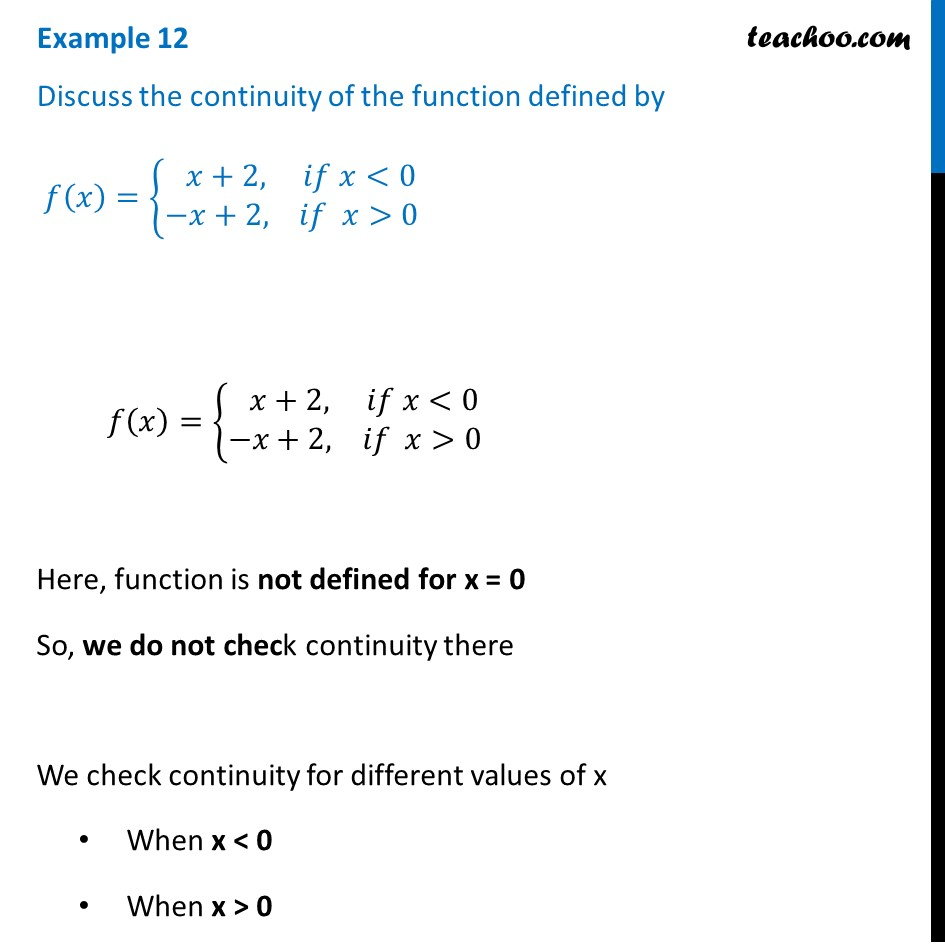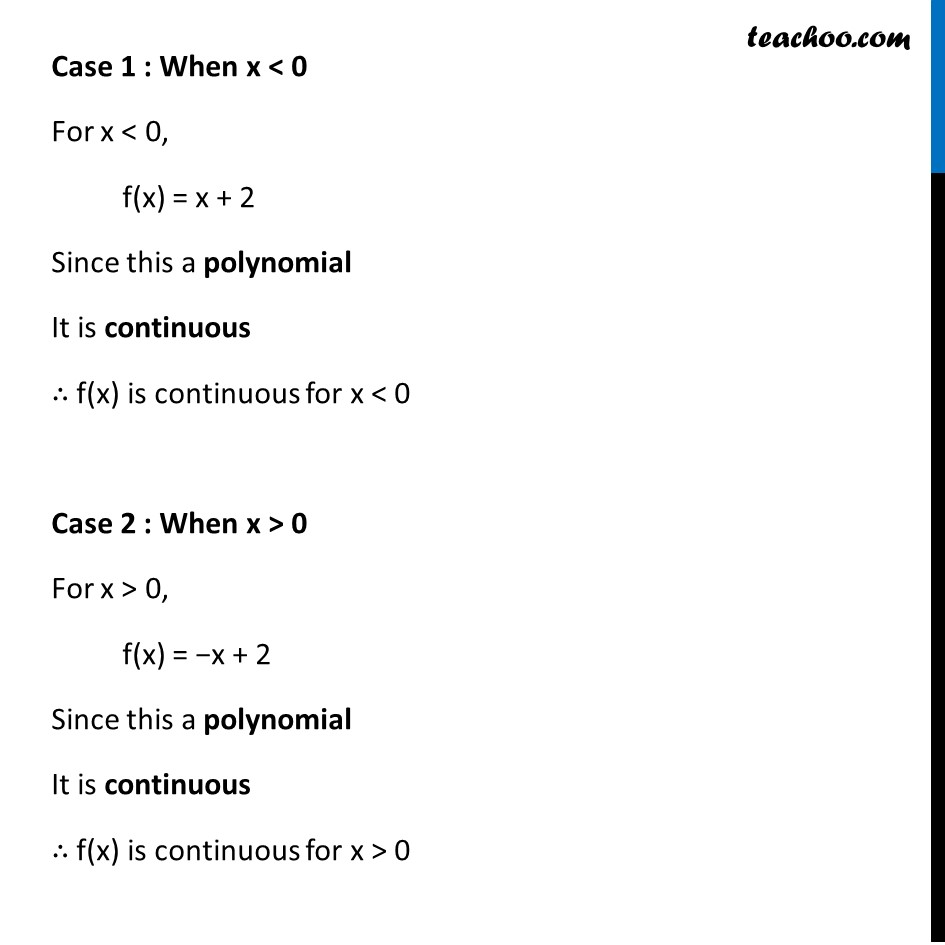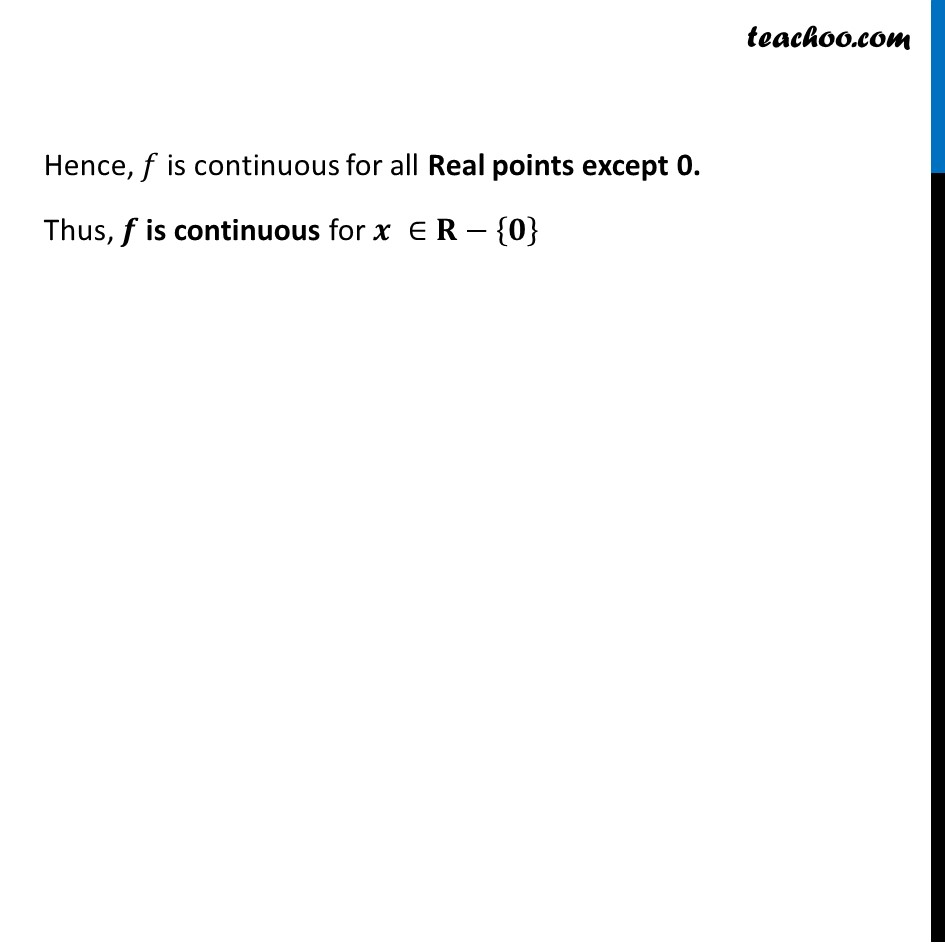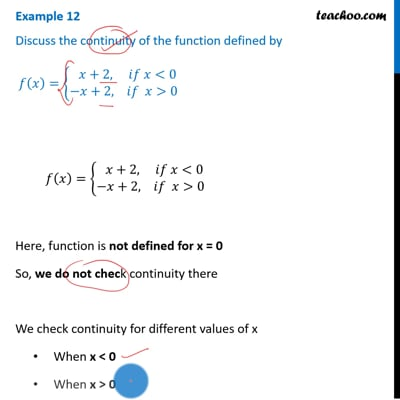Examples

Chapter 5 Class 12 Continuity and Differentiability
Serial order wiseThis video is only available for Teachoo black users

Solve all your doubts with Teachoo Black (new monthly pack available now!)

### Transcript

Example 12 Discuss the continuity of the function defined by 𝑓(𝑥)={█(& 𝑥+2, 𝑖𝑓 𝑥<0@&−𝑥+2, 𝑖𝑓 𝑥>0)┤ 𝑓(𝑥)={█(& 𝑥+2, 𝑖𝑓 𝑥<0@&−𝑥+2, 𝑖𝑓 𝑥>0)┤ Here, function is not defined for x = 0 So, we do not check continuity there We check continuity for different values of x When x < 0 When x > 0 Case 1 : When x < 0 For x < 0, f(x) = x + 2 Since this a polynomial It is continuous ∴ f(x) is continuous for x < 0 Case 2 : When x > 0 For x > 0, f(x) = −x + 2 Since this a polynomial It is continuous ∴ f(x) is continuous for x > 0 Hence, 𝑓 is continuous for all Real points except 0. Thus, 𝒇 is continuous for 𝒙 ∈𝐑−{𝟎}